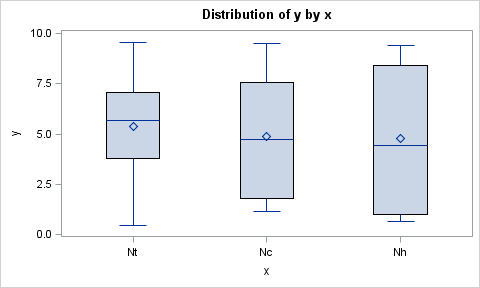## output order in boxplot

I'd like to use boxplot to make comparisons between three variables, say, Nh, Nt, Nc. I'd like to see the three boxplots side by side in the order: Nh, Nt, Nc. But the output displayed the boxplots in the order: Nc, Nh, Nt (alphabetically). How can I change the order to the one I want? Thanks!

Andrew

6 REPLIES 6Jay54
Meteorite | Level 14

## output order in boxplot

With the SGPLOT procedure (SAS 9.2), you can set DISCRETEORDER=data on the XAXIS statement to get data order.```data box;
do x='Nh', 'Nt', 'Nc';
do i=1 to 10;
y=10*ranuni(2);
output;
end;
end;
run;

ods listing;
ods graphics / reset width=5in height=3in imagename='BoxOrder';
proc sgplot data=box;
vbox y / category=x;
xaxis display=(nolabel) discreteorder=data;
run;
```

## output order in boxplot

Sorry I didn't make it clear. First, I'd like to use proc boxplot. Second, I'd like to control the order of the boxes in the boxplots. Not necessarily Nh, Nt, Nc, but maybe Nt, Nc, Nh. Would you please tell me how to do that using proc boxplot? Thanks.

## Re: output order in boxplot

You could manipulate your data so that the values of your boxes are in alphabetical order, and then create a format so that what displays is still the meaningful names, like 'Nt', 'Nc', and 'Nh'. For example:

data box;

do x='V3', 'V1', 'V2';

do i=1 to 10;

y=10*ranuni(2);

output;

end;

end;

run;

proc format;

value \$myboxval

'V1' = 'Nt'

'V2' = 'Nc'

'V3' = 'Nh';

run;

proc sort data=box;

by x;

run;

ods listing;

ods graphics / reset width=5in height=3in imagename='BoxOrder';

footnote '';

proc boxplot data=box;

format x \$myboxval.;

plot y*x=i;

run;## output order in boxplot

In the existing data set, the order is Nh, Nt, Nc. I know in proc freq, one can use "order=data" but not in proc boxplot. I don't want to restructure the data set. How can I solve the problem?Ksharp
Super User

## output order in boxplot

You can pad white blank before value of variables to customize your order.

Make sure that your variable has long enough to hold these white blanks.

```data box;
length x \$ 8;
do x='Nt', 'Nc', 'Nh';
do i=1 to 10;
y=10*ranuni(2);
output;
end;
end;
run;
data box;
set box;
if x='Nt' then x='  Nt';
else x='Nc' then x=' Nc';
run;

ods listing;

ods graphics / reset width=5in height=3in imagename='BoxOrder';

footnote '';

proc boxplot data=box;

plot y*x=i;

run;

```

Ksharp

## output order in boxplot

Add a dummy variable to the data set that has the values 1, 2, ...,k, where k is the number of categories. Then use a user-defined format as shown by

@Joeldw to map the dummy variable to the categorical values.

Discussion stats
• 6 replies
• 7051 views
• 0 likes
• 5 in conversation